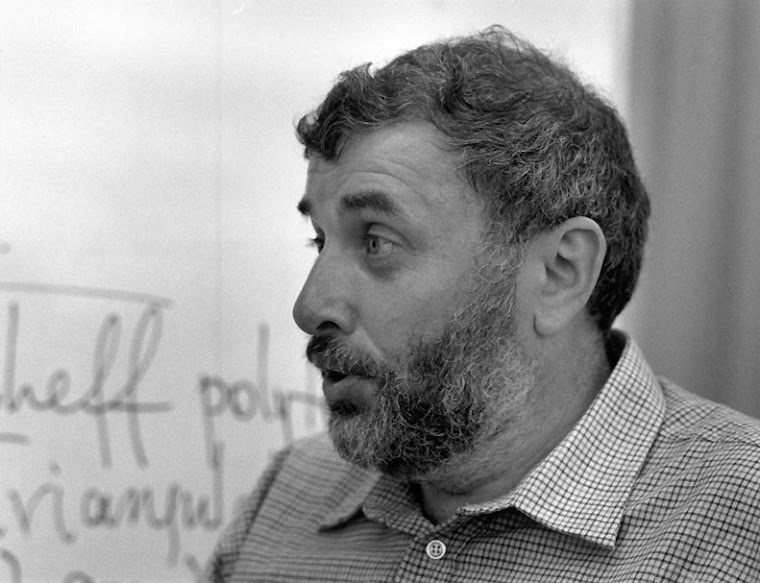## Wednesday, May 6, 2015

### Юноше, обдумывающему житьё

Tanya Khovanova posted a list of problems that Andrei had considered important for undergraduate students to think through and solve by themselves.

Problem 1. Let G be a finite group of order |G|. Let H be its subgroup, such that the index (G:H) is the smallest prime factor of |G|. Prove that H is a normal subgroup.

Problem 2. Consider a procedure: given a polygon in a plane, the next polygon is formed by the centers of its edges. Prove that if we start with a polygon and perform the procedure infinitely many times, the resulting polygon will converge to a point. In another variation, instead of using the centers of edges to construct the next polygon, use the centers of gravity of k consecutive vertices.

Problem 3. Find numbers an such that 1 + 1/2 + 1/3 + … + 1/k = ln k + γ + a1/k + … + an/kn + …

Problem 4. For x1 not equal to zero, let xk = sin xk-1. Find the asymptotic behavior of xk.

Problem 5. Calculate the integral from 0 to 1 of x−x over x with the precision 0.001.